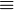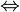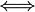# Logical equivalence facts for kids

Kids Encyclopedia Facts

In logic and mathematics, two statements are logically equivalent if they can prove each other (under a set of axioms), or have the same truth value under all circumstances. In propositional logic, two statements are logically equivalent precisely when their truth tables are identical. To express logical equivalence between two statements, the symbols$\equiv$,$\Leftrightarrow$ and$\iff$are often used.

For example, the statements "A and B" and "B and A" are logically equivalent. If P and Q are logically equivalent, then the statement "P if and only if Q" is a tautology.

## Related pagesLogical equivalence Facts for Kids. Kiddle Encyclopedia.